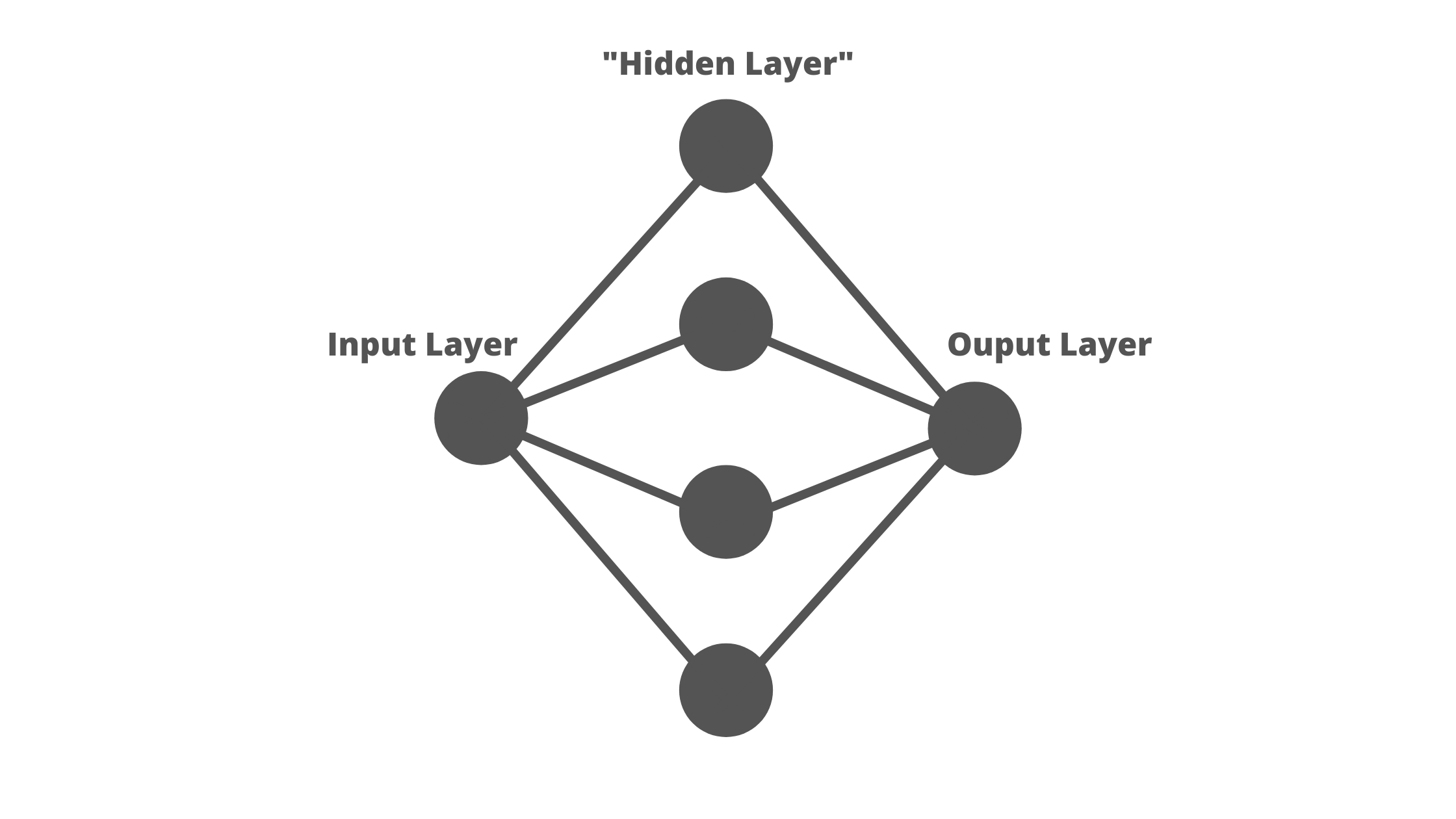# What does a deep learning neural network look like? — For Beginners

Original article was published by Joshua Potts on Artificial Intelligence on MediumOkay, so let’s jump right into our visualization with an extremely simple and minimalistic neural network.

What you’re seeing here looks super basic. This is a neural network with one input neuron, one output neuron and a single “hidden layer” with four neurons. You can think of this hidden layer as the black box, the thinking zone, where the patterns will be spotted.

# The Input Layer

The input layer is where we enter our data. When you’re training the network, you’ll put your sample data in here, but it’s also where the “real scenario” data goes when you actually want the network to solve a problem for real. In our model, it’s just a single neuron. Very simple. Usually each input neuron will have a value between 0 and 1, or -1 and 1 — a float value, for the programmers out there.

Or, depending on your problem, your input value could function as a boolean, or “true or false” essentially. For example, you might have a value for whether the traffic lights are red or green — 0 for red, and 1 for green.

By the way, you’re not limited to one input neuron. Neural networks that analyse images can have millions of input neurons, each representing a pixel.

A good way to understand the input layer is to think of it as the box where you put the data you know about your problem. The neural network’s job is to take this data and solve the problem, then fire out an answer.

# The Hidden Layer(s)

Nowadays there are very few deep learning programs where we manually tune the hidden layer, or even know what the values are in it. This is because when the neural network trains itself, by finding its mistakes and calculating where it went wrong, it will go back to the hidden layers, tweak them, and run another set of input data through them — continually making changes until it has a unique set of values for each connection and each neuron to solve a specific problem as accurately as possible.

So what exactly is the hidden layer? This elusive layer of neurons in the middle of our network is where most of the “brain work” of our neuron happens. By having at least 1 hidden layer, you drastically increase the number of connections in your network, and thereby the number of “weights and biases” that can be tweaked when your network is learning to solve a problem. When you change these values in different ways, the neurons “talk” to each other in different ways, leading to different calculations and ultimately, a clever output targeted towards your problem.

Again, you can have as many hidden layers as you have computational power for, but you can accomplish a huge deal with just one layer, and generally having more than about six doesn’t offer many benefits. I’ll explain this in a future article.

# Output Layer

This layer is where we get our answer, and where the final calculations leading to our outputs happen. You can have as many output neurons as you wish for (realistically-speaking, of course). On the first try of your neural network, all the “weights and biases” are randomized, so statistically the first set of outcomes will be very wrong. That’s why we train neural networks using “backpropagation”. Summarising, this is where the network looks at the answer it calculated, compares it to the correct answer it should have calculated, and goes back to find where it went wrong in its calculations. It then tunes its weights and biases and tries again. Repeatedly until it accumulates a good level of accuracy.

I’ll go into weights and biases in my next article, and explain how they affect the neurons and connections in your network, and why they allow neural networks to perform clever calculations to find outputs. Looking forward to seeing you there!